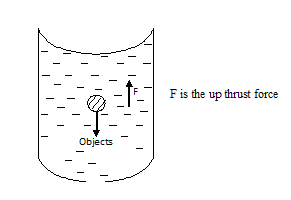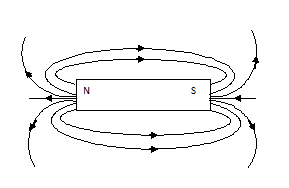# PHY1: FORCES

##### This unit is about force and the types it has.

FORCES

Definition

It is something that changes the body’s state of rest or uniform motion in a straight line.

EFFECTS OF FORCES

– It causes moving bodies to reduce speed or stop moving

– It deforms objects

– It causes a body at rest to move

– It causes increment in the spies of moving objects

– It causes a body to change direction

Force is measured in new tons [N]

THIS VIDEO BRIEFLY DESCRIBES FORCE

CALCULATION OF FORCE

Force is calculated by using formulae

F = ma, where m is the mass of the body and a is the acceleration of the body.

Note:

the mass should be measured in kg;

EXAMPLES

Find the force acting on a body of mass 4kg  moving  with acceleration of 2m/s2

F = m x a

= 4 x 2

= 8N

What force does a 620g body exert when with an acceleration of 2m/s2?

F = m x a

620g to kg=(620/1000)kg

= 0.6 x 2

= 1.24N

TYPES OF FORCE

1. Up thrust force
2. Gravitational force
• Friction force
1. Centripetal force
2. Magnetic force
3. Molecular force
• Electrostatic force
• Elastic force

GRAVITATIONAL FORCE

This is a force the earth exerts on bodies by putting them towards its centre

The gravitational force is experienced in the following;-

– climbing a hill

– A dry leaf falling from a tree

– An object thrown up in space / our falling down etc

– jumping

This force is experienced in the gravitational force.

WEIGHT

This is the force a body exerts on anything that freely supports it in the gravitational field.

Weight is obtained from; w = mg where m is mass of the body and g the acceleration due to gravity

COMPARISON BETWEEN MASS AND WEIGHT

 Mass Weight 1.      Quality of mattering body Force a body exerts on anything that freely supports in the grow field 2.      Mass is constant Changes from place to place 3.      Mass is scalar quality Weight is a sector quality 4.      Measured using beam balance Measured using spring balance 5.      Measured in kilo grams Measured in newtons

Note:

Weight values changes slightly from place to place on earth due to;

1. The shape of the earth i.e. the earth bulge at the equator
2. The earth’s rotation

CALCULATION OF WEIGHT

Weight is measured in newtons.

W = mg, the acceleration of gravity is constant on earth: g =10 m/s2

EXAMPLES

1. Calculate the weight of a body with mass of 0.8kg

W=mg

= 0.8 x 10

= 8N

1. Mass of 500g

W= mg

= 0.5 x 10

= 5N

1. Calculate the mass of a body whose weght is 20N

W= mg

20N = M x 10

= 20/10 =10m/10

M = 2kg

NOTE:

1kg = 10N

1. What is the weight of a 1kg body

W= mg

=1 x 10

= 10N

1kg = 10N

EXERCISE

Convert the following;-

1. 2kg to newtons

1kg = 10N

0.2 kg = 0.2 x 10

= 2N

1. 4 N to kg

10N = 1kg

1N =  1/10kg

4N=1/10kg x4

=0.4kg.

1. 0043kg to N

1kg = 10N

0.0043kg =( 10×0.0043)N

= 0.043N.

CENTRIPETAL FORCE

This is the force that keeps bodies moving in a circular path. This force moves bodies to the centre of the circle.EXAMPLES

1. A car negotiating in a corner
2. The moon in a bit with a planet
• Pendulum

ELECTROSTATIC FORCE

Force extorted on a charged body in an electric field. It may be repulsive or attractive.

– Like charge repel and unlike charges attract

– The region around the charged body or a charge where electric fore is experienced.

MOLECULAR FORCE

Force of attraction between molecules of same substances. These include;-

• cohesion

Force of attraction between molecules of the same substance

Force of attraction between molecules of different substances

UP THRUST FORCE

Upward force experienced by objects in fluidsMAGNETIC FORCE

This is the force extorted by a magnet on a material. It is either repulsive or attractive

A magnet is a piece of metal or alloy which has ability to repel. The north and South Pole

A magnetic pole is the area near the ends of a magnet were the resultant  attractive force appears to concentrate.A magnetic field is the area around the magnet where the magnetic force is applied.

Magnetism is the ability of a metal or alloy. [Magnet] to attract or repel other materials.

We use lines and arrows to show a pattern of a magnetic field. The arrows indicated the direction in which the magnetic force is acting.

When drawing magnetic field patterns, the following as properties of magnetic field lines must be considered;

– The lines run from the north to south pole so arrows should be drawn showing the direction of north to south.

– Magnetic field lines do not meet i.e. they are parallel.

Note

Like poles repel where as unlike poles attract

SKETCHES OF MAGNETIC FIELD PATTERNS

A magnetic field due to a single isolated magnet.Two magnets with unlike poles nearTwo magnets with like poles near

X is the neutral point

The neutral point in a magnetic field represents the region where the resultant magnetic flux is 0.

### ASSIGNMENT : FORCES assignmentMARKS : 10  DURATION : 1 week, 3 days

SEE ALL
•YOU